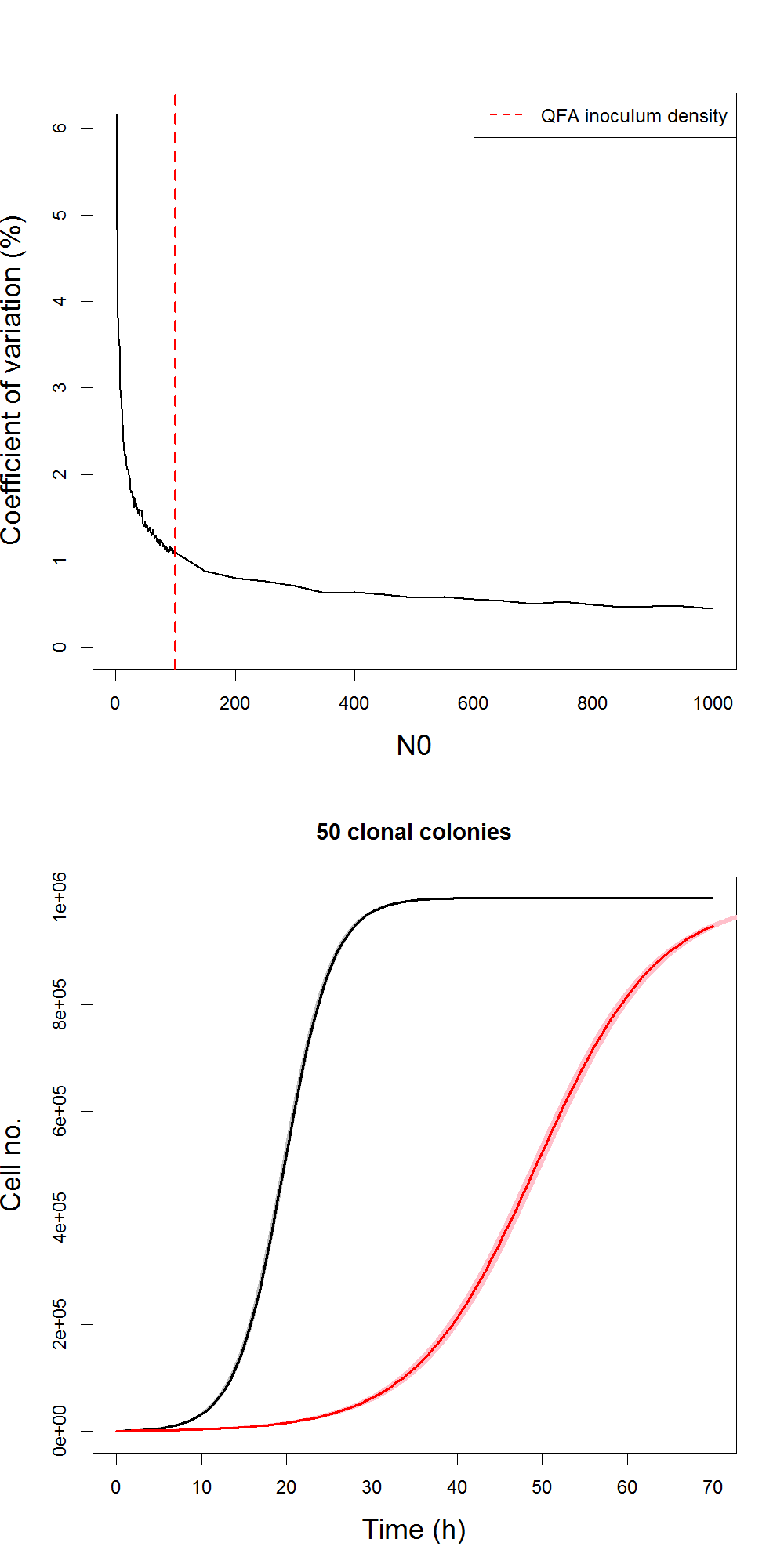# A discrete stochastic logistic model of cell lineages

Conor Lawless
SBSSB February 2013

http://lwlss.net/talks/discstoch

### Overview

• Datasets and motivation for model
• Logistic model - population dynamics
• Logistic model - mass action chemical kinetics
• Discrete stochastic logistic model (DSLM) simulation with Gillespie algorithm
• Vectorised simulation of DSLM
• Hybrid simulation of DSLM
• Parameter inference for DSLM
• Future work

### Datasets and motivation for model

µQFA data (heterogeneity)

Single cultures (survivors)Heterogeneity from stochastic processes during first divisions?

### Logistic model - population dynamics

Proposed by Verhulst in 1838 as an improvement to the Malthusian exponential model for describing population growth.

$\frac{dN}{dt} = r N\left ( 1-\frac{N}{K} \right )$

Continuous, deterministic ODE describing exponential growth with a density dependence term

$N(t) = \frac{K N_0 e^{rt}}{K + N_0 \left( e^{rt} - 1\right)}$

Analytical solution makes simulation and inference easier### Logistic model - mass action chemical kinetics

Reaction

$Cell + Nutrient\rightarrow 2 \hspace{0.25em}Cell$

Rate expression

$r[Cell][Nutrient]$

ODE representation

$\frac{d[Cell]}{dt} = r[Cell][Nutrient]\\ \frac{d[Nutrient]}{dt} = -r[Cell][Nutrient]$

Defining concentration

$[Cell]=\frac{N_{Cell}}{K}\\ [Nutrient]=\frac{N_{Nutrient}}{K}$

Conservation of mass

$\frac{dN_{Cell}}{dt}+\frac{dN_{Nutrient}}{dt}=0\\ N_{Cell}+N_{Nutrient}=K$

Familiar form

$\frac{d[Cell]}{dt} = r[Cell][Nutrient]\\ \Rightarrow \frac{1}{K}\frac{N_{Cell}}{dt}=r\frac{N_{Cell}}{K}\frac{(K-N_{Cell})}{K}\\ \Rightarrow \frac{dN_{Cell}}{dt} = r N_{Cell}(1-\frac{N_{Cell}}{K})$

### Logistic model - mass action chemical kinetics

• Describe model in SBML
• Explicit assumptions
• Perfect mixing
• Molecular interactions in gaseous phase
• Mechanical agitation in liquid phase
• Diffusion of proteins etc. within cells
• Random interactions in animal populations
• Reaction hazard depends only on current state (& parameters)
• Reaction hazard function

### Discrete stochastic logistic model (DSLM) simulation with Gillespie algorithm

Hazard function

$h(N,r,K) = r N\left ( 1-\frac{N}{K} \right )$

Simulate time between cell divisions

$\delta t_i \sim{ Exp(h(N_i,r,K))}$

Gillespie algorithm

\begin{align} t_i &= t_{i-1} + \delta t_{i-1}\\ N_i &= N_{i-1} + 1\\ \end{align} \forall i=1,...,K-N_0

Assume no cell death (look for differences in rate of progression of cell cycle).

### Vectorised simulation of DSLM

simDSLogistic=function(K,r,N0){
# Unusually, for this model, we know the number of events a priori
eventNo=K-N0
# So we can just generate all required random numbers (quickly) in one go
unifs=runif(eventNo)
# Every event produces one cell and consumes one unit of nutrients
clist=(N0+1):K
# Simulate time between events by generating
# exponential random numbers using the inversion method
dts=-log(1-unifs)/(r*clist*(1-clist/K))
return(data.frame(t=c(0,cumsum(dts)),c=c(N0,clist)))
}

### Vectorised simulation of DSLM• Variability higher for slow-growing strains
• Individual curves continuous & deterministic after initial divisions
• One simulation of realistic population size ~ 600 ms
• Fast, but not fast enough for inference

### Hybrid simulation of DSLM

simCellsHybrid=function(K,r,N0,NSwitch,detpts=100){
# Every event produces one cell and consumes one unit of nutrients
if(NSwitch>N0){
# Unusually, for this model, we know the number of events a priori
eventNo=NSwitch-N0
# So we can just generate all required random numbers (quickly) in one go
unifs=runif(eventNo)
clist=(N0+1):NSwitch
# Time between events
dts=-log(1-unifs)/(r*clist*(1-clist/K))
# Absolute times
ats=cumsum(dts)
tmax=max(ats)
}else{
clist=c()
ats=c()
tmax=0
}
# Switch to discrete deterministic logistic function
clistdet=seq(NSwitch+(K-NSwitch)/detpts,K,(K-NSwitch)/detpts)
tsdet=log((clistdet*(K - NSwitch))/((K - clistdet)*NSwitch))/r
return(data.frame(t=c(0,ats,tmax+tsdet),c=c(N0,c(clist,clistdet))))
}

~0.5 ms per simulation, compared to ~0.1 ms for deterministic simulation, 600 ms for DSLM

### Hybrid simulation of DSLM

Switch to deterministic simulation: which $N$?

Coefficient of variation in time to reach $N=\frac{K}{2}$

Lowest inoculum density giving high precision in QFA cell density estimates?

1,000 cells gives COV < 1%### Likelihood free parameter inference

• Synthetic dataset (unfortunately)
• Fast hybrid DSLM Simulation in "pure" R
• Particle MCMC inference following example in Darren's book & blog post
• Uninformative priors for $r$ and $K$ ($N_0=1$)
• 100,000 updates with thinning of 100
• Marginal likelihood particle filter: 10 particles
• No autocorrelation problems
• ~20 min per timecourse (a bit slow)### Future work

• Develop image analysis software for photography data
• Gather data...
• Calibration curve: nonlinear relation between observation (colony area & colour) and cell count
• Inference with multiple timecourses (multiple colonies)
• Individual based simulation model
• Realistic interdivision time distributions
• Replicative ageing (protein accumulation)
• Telomerase negative cells: telomere shortening & senescence

### µQFA data - microscopy

Clonal microcolonies growing in isolation, merging to form a culture

Video duration ~10 hours

### Single colony data - photography

Clonal colonies growing in isolation until nutrients are exhausted.

Video duration ~3 days